Thursday , July 7 2022# NCERT 5th Class (CBSE) Mathematics: Polygons And Circles

### Construction of Squares and Rectangles

(a) you can draw a square or a rectangle with the help of a set square.

A set square is a drawing instrument with a right angle. We use it with a ruler to get sharp corners of squares and rectangles.

Draw a rectangle ABCD with sides 4 cm and 6 cm.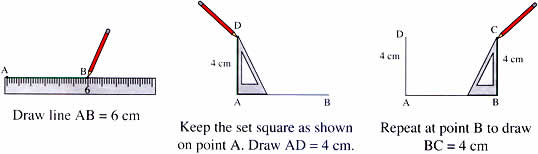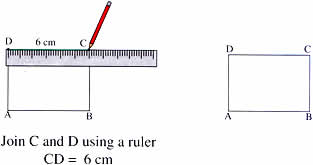(b) You can also use a protractor to draw squares and rectangles.

Draw square PQRS with sides of 5 cm.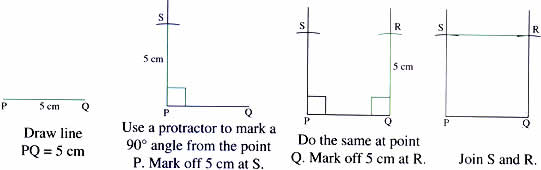## CIRCLES

What shape do these pictures remind you of?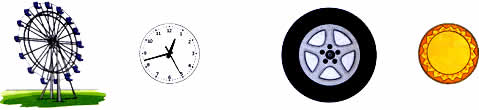All these objects remind us of the shape of a circle.

A circle is a simple closed curve. It is made of a curved line. ‘O’ is the Center of the circle. We can name the circle by its center. This is circles O.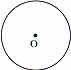This is the radius of the circle. The radius is a line segment that runs between the circle and its center. This is  radius OP. We can have several radii all of the same length starting from O going to other points on the circle. This is because all the points on the circle are at an equal distance from the center.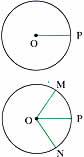## चुनौती हिमालय की 5th NCERT CBSE Hindi Rimjhim Ch 18

चुनौती हिमालय की 5th Class NCERT CBSE Hindi Book Rimjhim Chapter 18 प्रश्न: लद्दाख जम्मू-कश्मीर राज्य में …